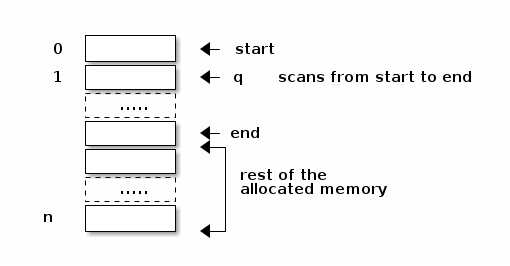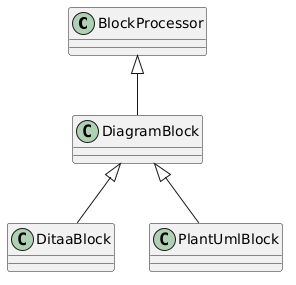Failed to generate image: Could not find the 'pdflatex' executable in PATH; add it to the PATH or specify its location using the 'pdflatex' document attribute
\usepackage{tkz-euclide}
\usepackage{etoolbox}
\usepackage{MnSymbol}
% \usetkzobj{all}
\usetikzlibrary{angles,patterns,calc}
\usepackage[most]{tcolorbox}
\usepackage{pgfplots}
\pgfplotsset{compat=1.7}

~~~~
\begin{tikzpicture}
\tikzset{>=stealth}
% draw axises and labels. We store a single coordinate to have the
% direction of the x axis
\draw[->] (-4,0) -- ++(8,0) coordinate (X) node[below] {$x$};
\draw[->] (0,-4) -- ++(0,8) node[left] {$y$};

% special method of noting the position of a point

\draw[thick]
(0,0)
coordinate (O) % store origin
node[] {} % label
--
node[below left, pos=1] {$P(-\frac{\sqrt{2}}{2}, -\frac{\sqrt{2}}{2})$} % some labels
node[below right, midway] {$r$}
(P)
--
node[midway,left] {$y$}
(P |- O) coordinate (Px) % projection onto horizontal line through
% O, saved for later
--
node[midway, below] {$x$}
cycle % closed path

% pic trick is from the angles library, requires the three points of
% the marked angle to be named

pic [] {angle=X--O--P};
\draw[->,red] (5mm, 0mm) arc (0:-495:5mm) node[midway,xshift=-4mm,yshift=3.5mm] {$-495^\circ$};
% right angle marker
\draw ($(Px)+(0.3, 0)$) -- ++(0, -0.3) -- ++(-0.3,0);
\end{tikzpicture}